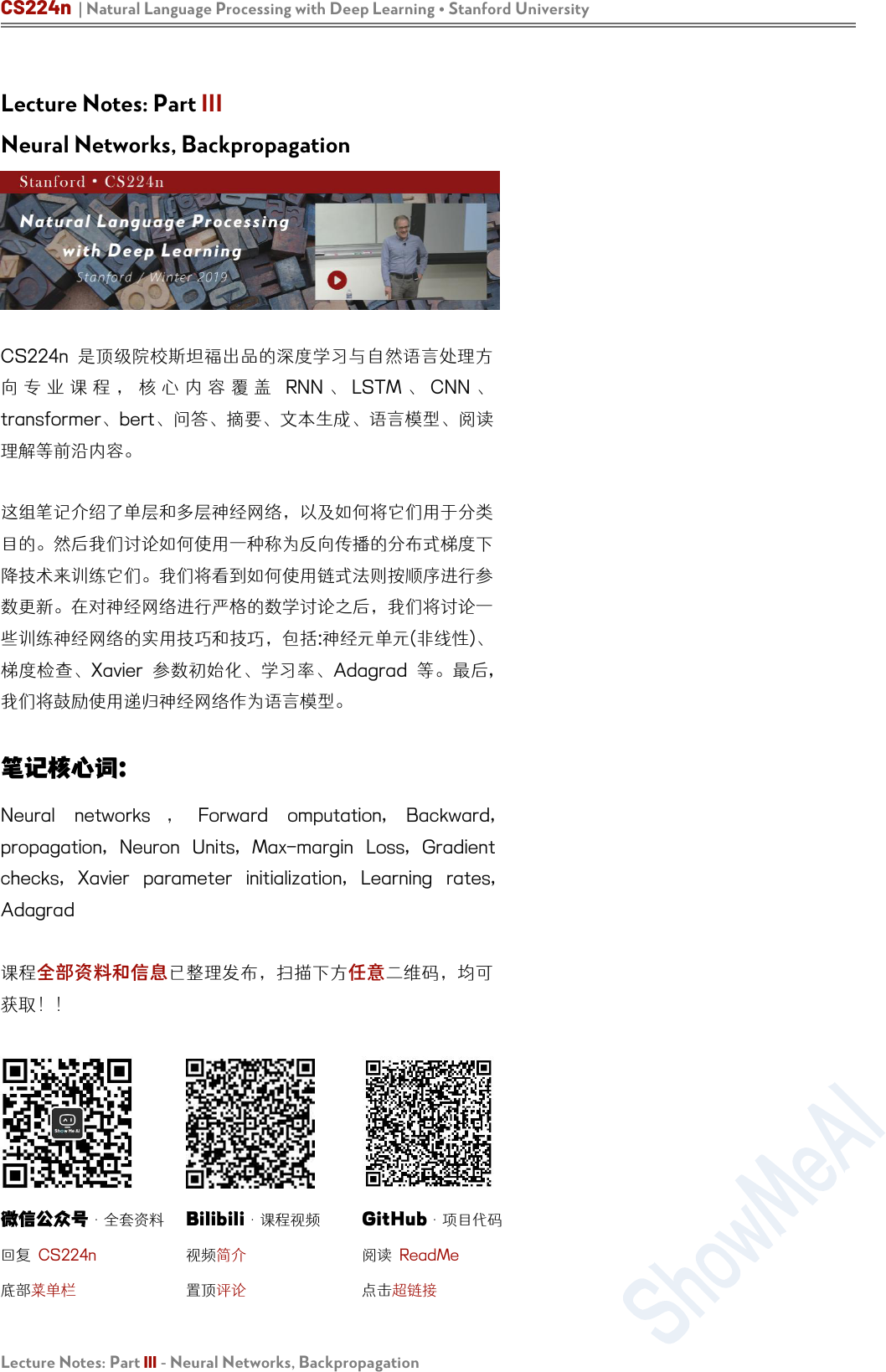CS224n | Natural Language Processing with Deep Learning Stanford University
Lecture Notes: Part III - Neural Networks, Backpropagation
Lecture Notes: Part III
Neural Networks, Backpropagation
CS224n
RNN LSTM CNN
transformerbert问答要、成、模型

Neural networks Forward omputation, Backward,
propagation, Neuron Units, Max-margin Loss, Gradient
checks, Xavier parameter initialization, Learning rates,

·全套资料

Bilibili
·课程视频

GitHub
·项目代码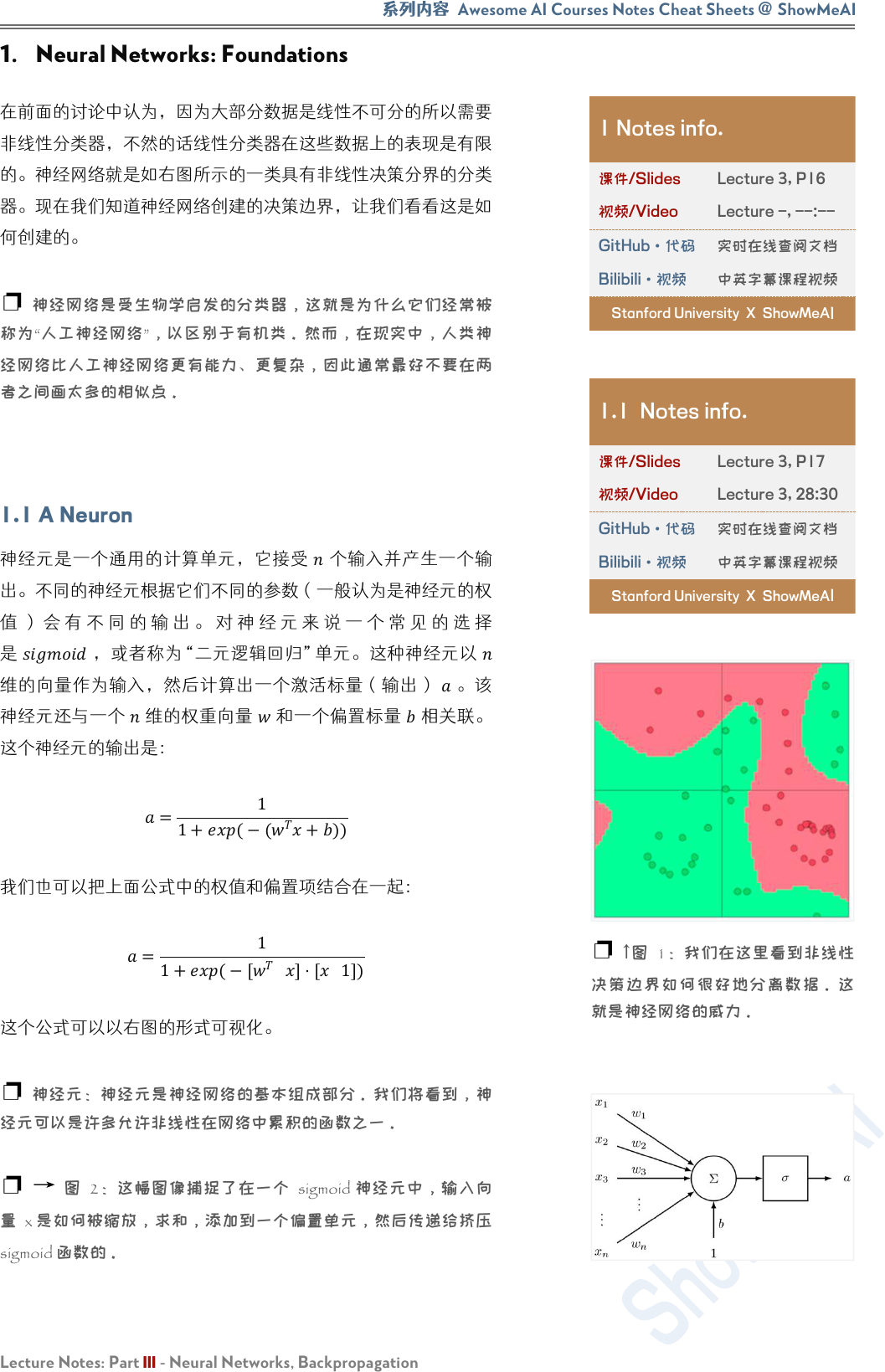Awesome AI Courses Notes Cheat Sheets
@
ShowMeAI
Lecture Notes: Part III - Neural Networks, Backpropagation
1. Neural Networks: Foundations

1.1 A Neuron



=
1
1+((
+))

=
1
1+([

][

1])

2 sigmoid
x 是如何被放，求和添加到一个偏单元，然后传给挤
sigmoid 函数的。
1 Notes info.

Lecture 3, P16

Lecture -, --:--
GitHub·代码

Bilibili·视频

Stanford University X ShowMeA
I
1.1 Notes info.

Lecture 3, P17

Lecture 3, 28:30
GitHub·代码

Bilibili·视频

Stanford University X ShowMeA
I
1线CS224n | Natural Language Processing with Deep Learning Stanford University
Lecture Notes: Part III - Neural Networks, Backpropagation
1.2 A Single Layer of Neurons

{w
(1)
,...,w
(m)
}
{b
1
,...,b
m
}

{a
1
,...,a
m
}
a
1
=
1
1+exp − w
(1)T
x+b
a
m
=
1
1+exp
− w
(m)T
x+b

σ z =
1
1+exp
z
1
1
1+exp
z
m
b=
b
1
b
m
m
W=
−w
(1)T
−w
(m)T
m×n

=+

a
1
a
m
=σ z =σ Wx+b

1.3 Feed-forward Computation


。但这么直觉

MuseumsinParisareamazing
1.2 Notes info.

Lecture 3, P18

Lecture -, --:00
GitHub·代码

Bilibili·视频

Stanford University X ShowMeAI
3
sigmoid

1.3 Notes info.

Lecture 3, P20

Lecture 3, 33:00
GitHub·代码

Bilibili·视频

Stanford University X ShowMeAIAwesome AI Courses Notes Cheat Sheets
@
ShowMeAI
Lecture Notes: Part III - Neural Networks, Backpropagation






1.2

×1

s=U
T
a=U
T
f(Wx+b)

1.4 Maximum Margin Objective Function


s
Not all museums in Paris

(
)

(
)
。然

>(
)>0

>

(
)
，否则为 0 。因此，我们的优化的目标函数现在为：
minimize J=max (s
c
s,0)

(
<)

<0)算。我们化目

minimize J=max (Δ+s
c
s,0)

=1
，让其他参数在优化过程中

x
20

W
8×20
b
8
U
8×1
s
1.4 Notes info.

Lecture 3, P33

Lecture -, --:00
GitHub·代码

Bilibili·视频

Stanford University X ShowMeAI
4图像CS224n | Natural Language Processing with Deep Learning Stanford University
Lecture Notes: Part III - Neural Networks, Backpropagation



minimize J=max (1+s
c
s,0)

=
(
+)
=
(+)
1.5 Training with Backpropagation Elemental
1.4 模型
0

(+1)
=
()

()

x
i

s

k

j

z
j
(k)

a
j
(k)

j
(k)

j
(k)
1
1
x
j
(k)
=z
j
(k)
=
a
j
(k)
W
(k)
k
k+1
Section 1.3
W
(1)
=
W W
(2)
=U
J=(1+
s
c
s)
W
14
(1)
W
14
(1)
z
1
(2)
a
1
(2)

a
1
(2)

W
1
(2)

Notes info.

Lecture 4, P12

Lecture 3, 57:00
GitHub·代码

Bilibili·视频

Stanford University X ShowMeAI
5 4-2-1
k j
()
，并产生激活输出
()Awesome AI Courses Notes Cheat Sheets
@
ShowMeAI
Lecture Notes: Part III - Neural Networks, Backpropagation


=−

=−1



(1)
。所以，



(1)
=

(2)
(2)


(1)
=

(2)
(2)

(1)
=
(2)

(2)

(1)
(2)

(2)


(1)
=
(2)

(2)

(2)

(2)


(1)
=
(2)
 
(2)

(2)

(2)


(1)
=
(2)
'
(2)

(2)

(1)
=
(2)
'
(2)


(1)
(1)
+
1
(1)
1
(1)
+
2
(1)
2
(1)
+
3
(1)
3
(1)
+
4
(1)
4
(1)
=
(2)
'
(2)

(1)
(1)
+
(1)

(1)
=
(2)
'
(2)
(1)
=
(2)
(1)
a
(1)
δ
i
(2)
a
j
(1)
δ
i
(2)
2
i

a
j
(1)
W
ij

2

W
14
(1)
1. 我们从
1
(3)
1 的误差信号开始反向传播。
2.
1
(3)
1
(3)

1
(3)
=1
3. 1
1
(3)

1
(2)
4. 现在
1
(2)

1
(3)
×
1
(2)
=
1
(2)
（在
1
(3)

1
(3)
）。因此在
1
(2)

1
(2)
5. 与第 2
1
(2)

1
(2)

1
(2)

'(
1
(2)
)
6. 因此在
1
(2)

1
(2)
)
1
(2)
，我们将其定义为
1
(2)
7.
4
(1)

14
(1)
6
W
ij
(1)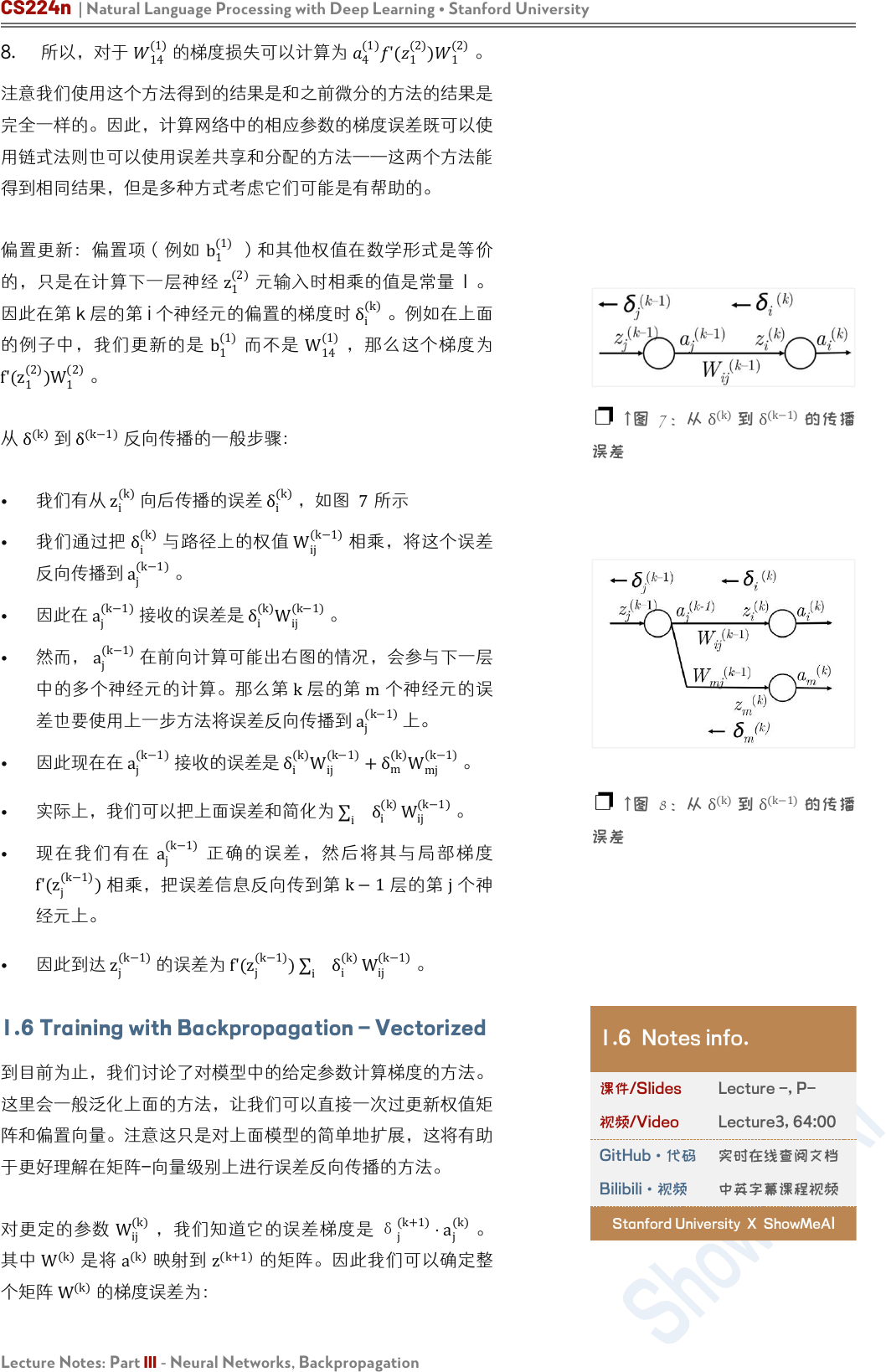CS224n | Natural Language Processing with Deep Learning Stanford University
Lecture Notes: Part III - Neural Networks, Backpropagation
8. 所以，对于
14
(1)

4
(1)
'(
1
(2)
)
1
(2)

b
1
(1)
z
1
(2)
1

i
(k)
。例如在上面
b
1
(1)
W
14
(1)
f'(z
1
(2)
)
W
1
(2)
δ
(k)
δ
(k−1)

z
i
(k)

δ
i
(k)
，如图 7所示
δ
i
(k)
W
ij
(k−1)

a
j
(k−1)

a
j
(k−1)

δ
i
(k)
W
ij
(k−1)

a
j
(k−1)

k
m

a
j
(k−1)

a
j
(k−1)

i
(k)
W
ij
(k−1)
+δ
m
(k)
W
mj
(k−1)

i
δ
i
(k)
W
ij
(k−1)
a
j
(k−1)
f'(z
j
(k−1)
)

k1
j

j
(k−1)

j
(k−1)
)
i
δ
i
(k)
W
ij
(k−1)
1.6 Training with Backpropagation Vectorized

W
ij
(k)
δ
j
(k+1)
a
j
(k)
W
(k)
a
(k)
z
(k+1)

W
(k)

7
δ
(k)
δ
(k−1)

8
δ
(k)
δ
(k−1)

1.6 Notes info.

Lecture -, P-

Lecture3, 64:00
GitHub·代码

Bilibili·视频

Stanford University X ShowMeA
I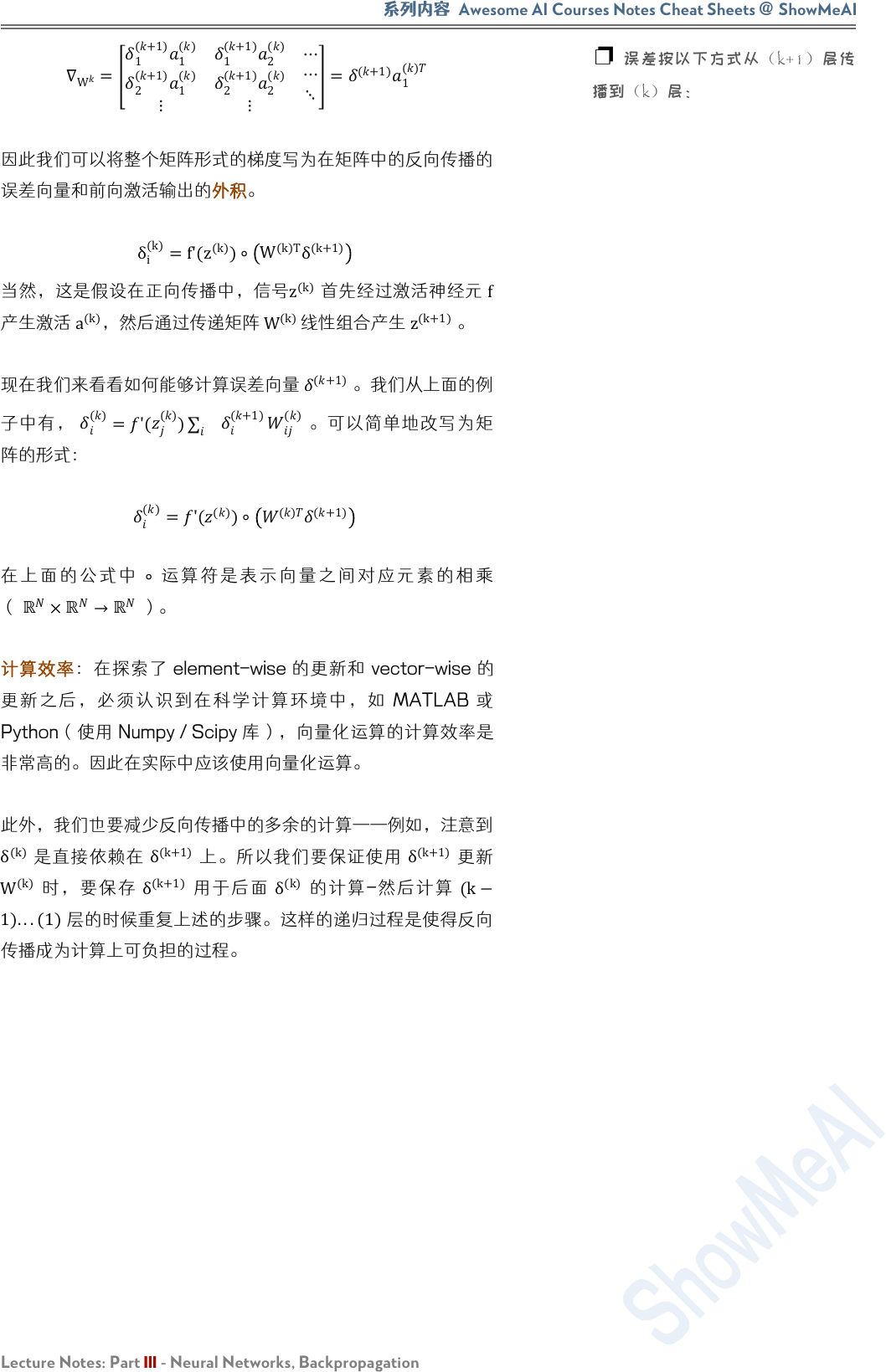Awesome AI Courses Notes Cheat Sheets
@
ShowMeAI
Lecture Notes: Part III - Neural Networks, Backpropagation
W
=
1
(+1)
1
()
2
(+1)
1
()
1
(+1)
2
()
2
(+1)
2
()
=
(+1)
1
()

δ
i
(k)
=f'(z
(k)
)
W
(k)T
δ
(k+1)

(k)

a
(k)
，然后通过传递矩阵
W
(k)

(k+1)

(+1)
。我们从上面的例
()
='(
()
)
(+1)

()

()
='(
()
)
()
(+1)
×
）。
element-wise vector-wise
MATLAB
Python使用 Numpy / Scipy

δ
(k)
δ
(k+1)

δ
(k+1)
W
(k)
δ
(k+1)
δ
(k)
- (k
1)...(1)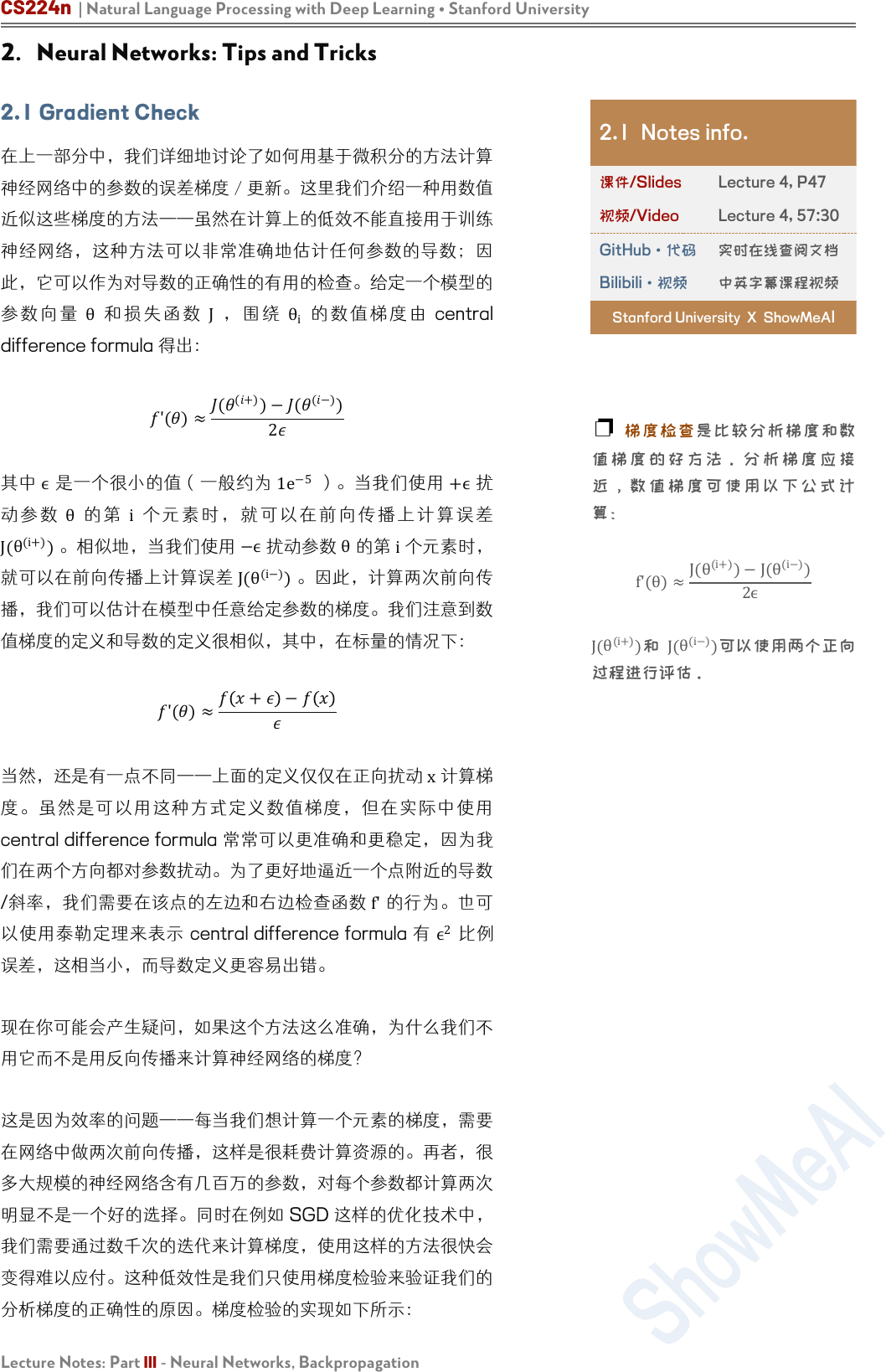CS224n | Natural Language Processing with Deep Learning Stanford University
Lecture Notes: Part III - Neural Networks, Backpropagation
2. Neural Networks: Tips and Tricks

θ
J
θ
i
central
difference formula 得出：
'()
(
(+)
)(
(−)
)
2
ϵ
1
e
−5

θ
i
J(
θ
(i+)
)。相似地，我们使
−ϵ

θ

(i−)
)。因

'()
+ −

x

central difference formula

/斜率我们 f'

ϵ
2

2.1 Notes info.

Lecture 4, P47

Lecture 4, 57:30
GitHub·代码

Bilibili·视频

Stanford University X ShowMeA
I

f'(θ)
J(θ
(i+)
)J(θ
(i−)
)
J(
θ
(i+)
) J(
θ
(i−)
)使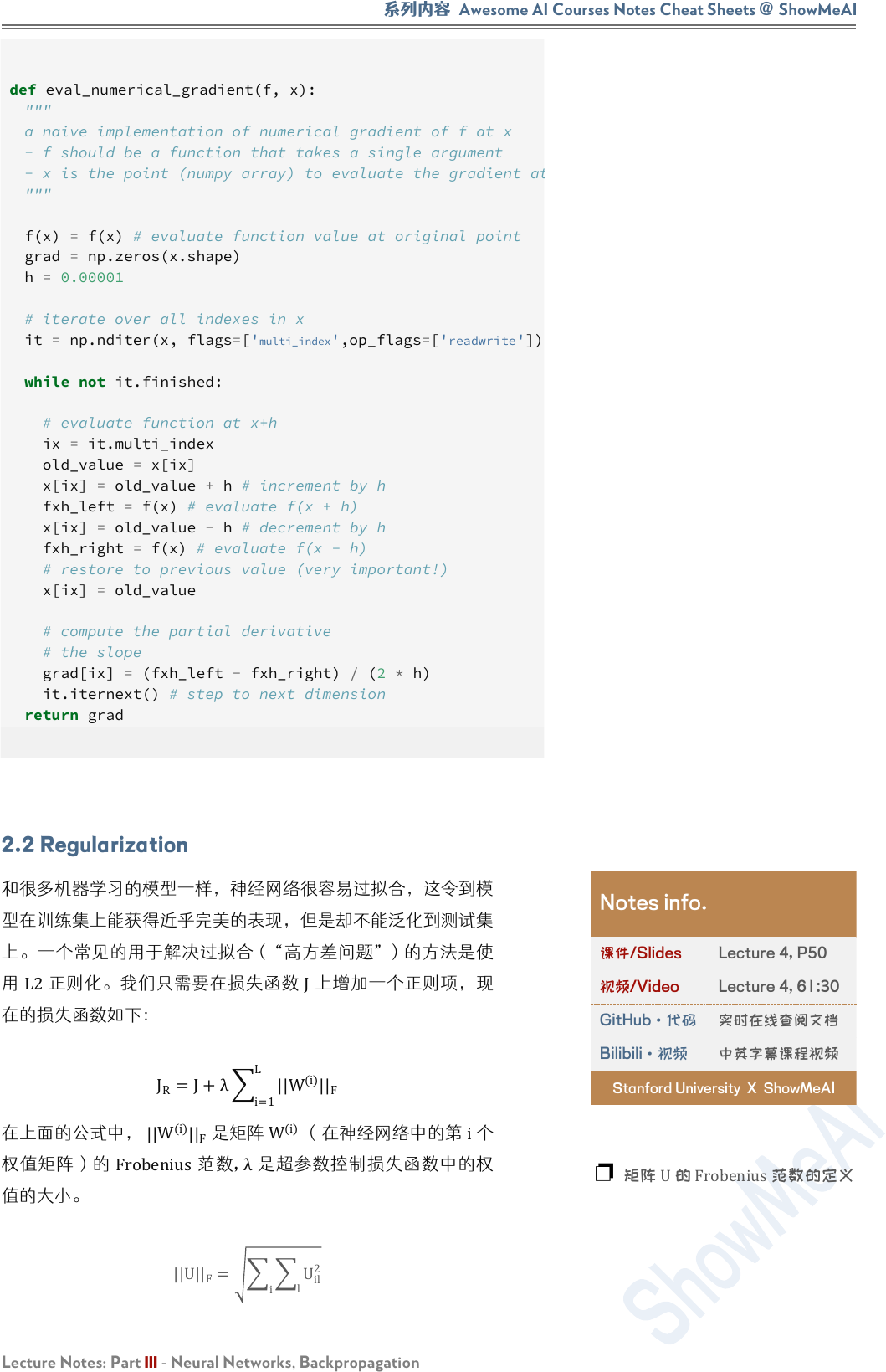Awesome AI Courses Notes Cheat Sheets
@
ShowMeAI
Lecture Notes: Part III - Neural Networks, Backpropagation
"""
a naive implementation of numerical gradient of f at x
- f should be a function that takes a single argument
- x is the point (numpy array) to evaluate the gradient at
"""
f(x) = f(x) # evaluate function value at original point
h = 0.00001
# iterate over all indexes in x
it = np.nditer(x, flags=['
multi_index
',op_flags=['
'])
while not it.finished:
# evaluate function at x+h
ix = it.multi_index
old_value = x[ix]
x[ix] = old_value + h # increment by h
fxh_left = f(x) # evaluate f(x + h)
x[ix] = old_value - h # decrement by h
fxh_right = f(x) # evaluate f(x - h)
# restore to previous value (very important!)
x[ix] = old_value
# compute the partial derivative
# the slope
grad[ix] = (fxh_left - fxh_right) / (2 * h)
it.iternext() # step to next dimension
2.2 Regularization

L2

J

J
R
=J+λ
i=1
L
||W
(i)
||
F

W
(i)
||
F

W
(i)
（在神经网络中的第 i
Frobenius
,
λ

||U||
F
=
i
l
U
il
2
Notes info.

Lecture 4, P50

Lecture 4, 61:30
GitHub·代码

Bilibili·视频

Stanford University X ShowMeAI

U
Frobenius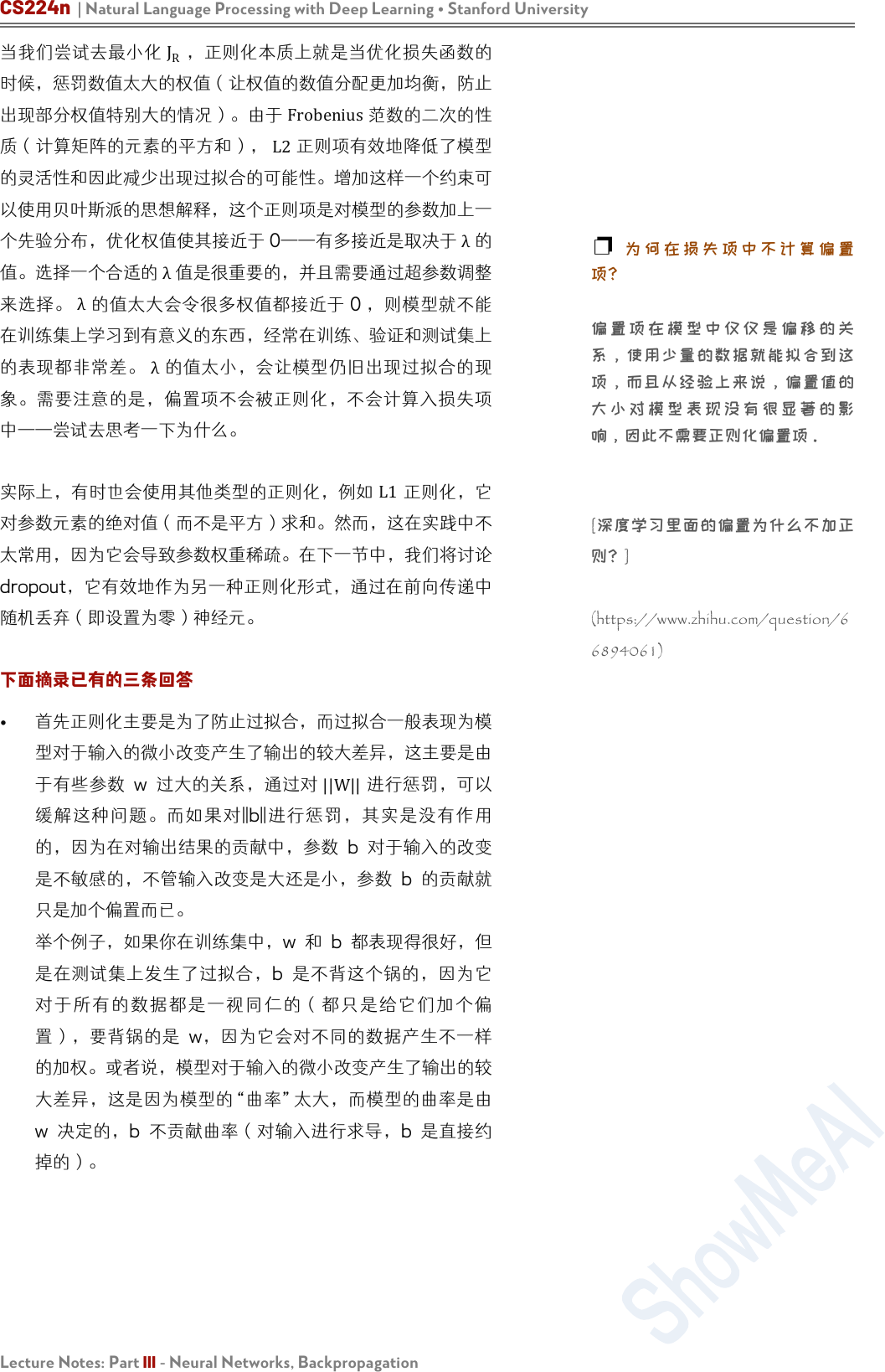CS224n | Natural Language Processing with Deep Learning Stanford University
Lecture Notes: Part III - Neural Networks, Backpropagation
J
R

Frobenius

L2

λ
0

λ
——尝试去思考一下为什么。

L1

dropout，它有效作为另一正则化形，通过在向传递中

w 系， ||W||
||b||

b
w

w b b

[

(https://www.zhihu.com/question/6
6894061)Awesome AI Courses Notes Cheat Sheets
@
ShowMeAI
Lecture Notes: Part III - Neural Networks, Backpropagation

W 线

L1

L1
dropout

Dropout

，而是对于该迭代，网络假定它们为 0 unit

2.3 Dropout
Dropout 一个非常强大正则化技术 Srivastava 在论
Dropout: A Simple Way to Prevent Neural Networks from
Overtting dropout

(1)

Dropout

dropout

Dropout applied to an artificial
neural network. Image credits to
Srivastava et al. Dropout
Srivastava 等人。】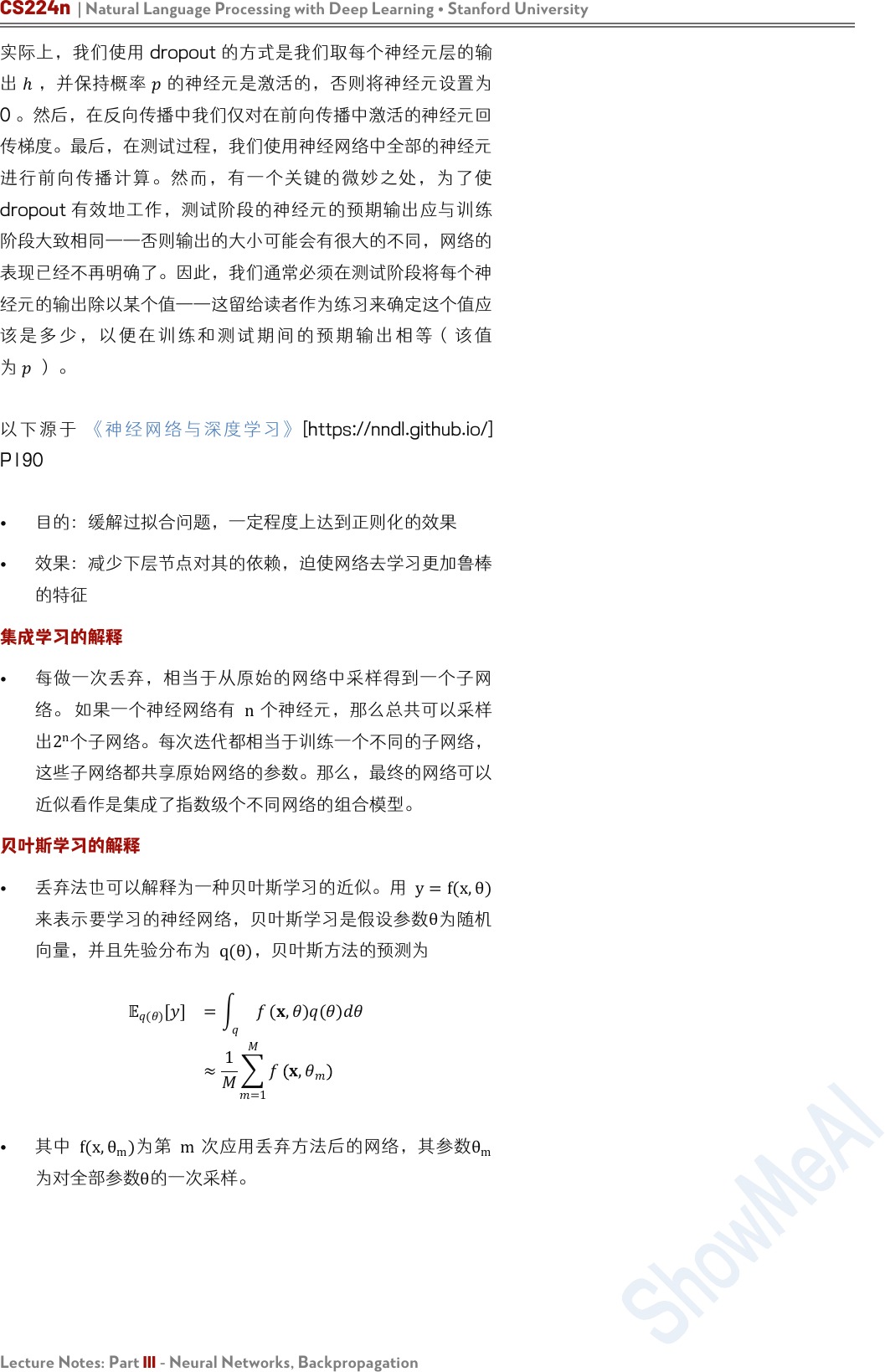CS224n | Natural Language Processing with Deep Learning Stanford University
Lecture Notes: Part III - Neural Networks, Backpropagation

0 。然后，在反向传播中我们仅对在前向传播中激活的神经元回

dropout

P190

n

2
n

y=f(x,θ)

θ

q(θ)
，贝叶斯方法的预测为
()
[] =
(,)()
1
=1
,
f(x,
θ
m
) m
θ
m

θ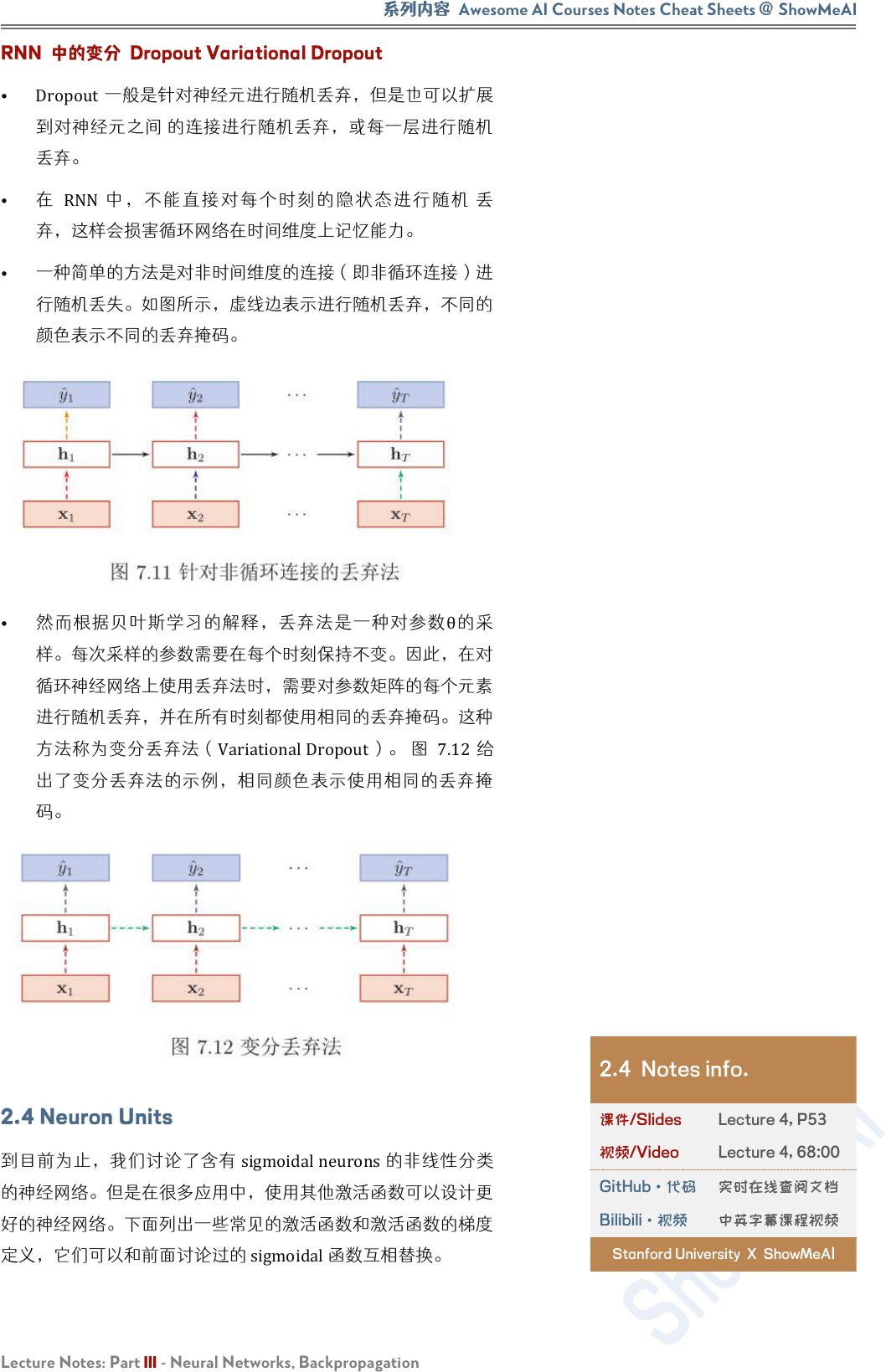Awesome AI Courses Notes Cheat Sheets
@
ShowMeAI
Lecture Notes: Part III - Neural Networks, Backpropagation
RNN 中的变分 Dropout Variational Dropout
Dropout一般是针对神经元进行随机丢弃，但是也可以扩展

RNN

θ

VariationalDropout 7.12

2.4 Neuron Units
sigmoidalneurons线

2.4 Notes info.

Lecture 4, P53

Lecture 4, 68:00
GitHub·代码

Bilibili·视频

Stanford University X ShowMeA
ICS224n | Natural Language Processing with Deep Learning Stanford University
Lecture Notes: Part III - Neural Networks, Backpropagation
Sigmoid：这是我们讨论过的常用选择，激活函数 σ为：
σ(z)=
1
1+exp(z)

σ(z)(0,1)
σ(z)的梯度为
σ
'
(z)=
−exp(−z)
1+exp(−z)
=σ(z)
1σ(z)
Tanh
tanℎ

sigmoid

tanℎ
sigmoid

tanℎ

sigmoid

ℎ()
()()
()+()
=2(2)

ℎ()


'
()=
()()
()+()
2
=1
2
()
Hard tanh ard tan
tanℎ

z

1

1ℎard tanℎ

ℎardtanℎ(z)=
−1 :z<1
z
:1z1
1 :z>1
ℎard tanℎ

ℎardtanℎ(z) =
1 :1z1
0 :otℎerwise
Soft sign soft sign数是另外种非线激活函数可以
tanℎ

softsign(z)=
z
1+|z|
soft sign

soft sign
'
z =
sgn(z)
1+z
2

sgn

z

rect'(z) =
1 :z>1
0 :otℎerwise
Figure 9: The response of a
sigmoid nonlinearity 9:sigmoid

Figure 10: The response of a
tanh nonlinearity 10:tanh 线

Figure 11: The response of a
hard tanh nonlinearity 11hard
tanh 非线性的响应】
Figure 12: The response of a
soft sign nonlinearity 12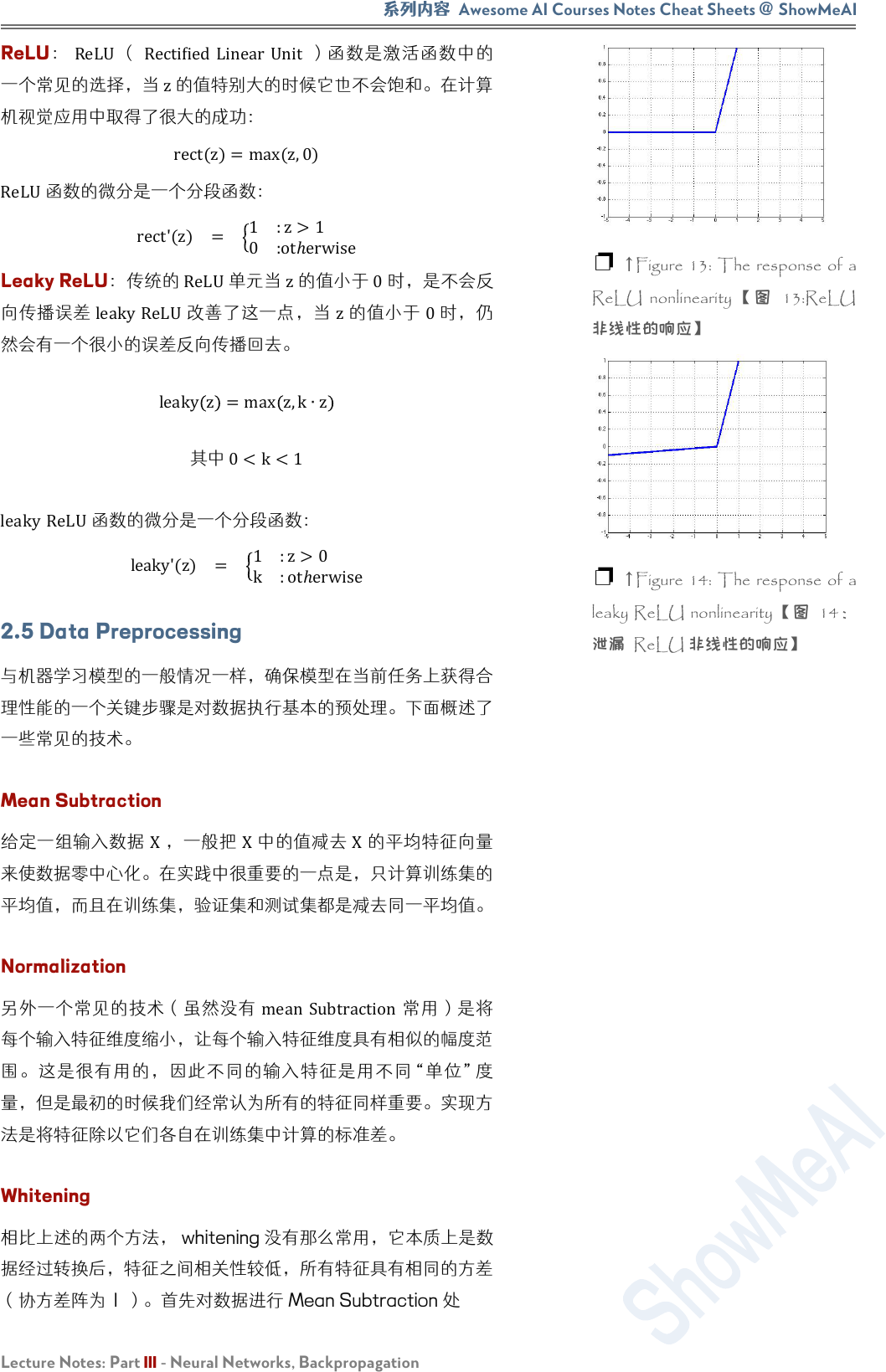Awesome AI Courses Notes Cheat Sheets
@
ShowMeAI
Lecture Notes: Part III - Neural Networks, Backpropagation
ReLU
ReLU
Rectiﬁed Linear Unit

rect(z)=max(z,0)
ReLU

rect'(z) =
1 :z>1
0 :otℎerwise
Leaky ReLU
ReLU

leaky ReLU
z
0

leaky
z
=max z,kz

0<k<1
leaky ReLU函数的微分是一个分段函数
leaky'(z) =
1 :z>0
k :otℎerwise
2.5 Data Preprocessing

Mean Subtraction
X
X
X

Normalization
mean Subtraction

Whitening

（协方差阵为 1 ）。首先对数据进 Mean Subtraction
Figure 13: The response of a
ReLU nonlinearity 13:ReLU

Figure 14: The response of a
leaky ReLU nonlinearity 14CS224n | Natural Language Processing with Deep Learning Stanford University
Lecture Notes: Part III - Neural Networks, Backpropagation
X'
X'
U
,
S
,
V
UX'
X'
U

S

0

2.6 Parameter Initialization
0
- Understanding
the difficulty of training deep feedforward neural networks
(2010), Xavier
training dynamics 验结 sigmoid
tanh
W
n
(l+1)
×
n
(l)

WU
6
n
(l)
+n
(l+1)
,
6
n
(l)
+n
(l+1)
n
(l)
W(fanin)
n
(l+1)
W(fanout)

0 。这种方法是尝试保持跨层之间的激活方差以及反向传播梯度

2.7 Learning Strategies

α

θ
new
=θ
old
α∇
θ
J
t
θ
α
——然而，在更快的收敛速度下并不能保证更快的收敛。实际

Figure 15: Here we see that
updating parameter w2 with a large
learning rate can lead to divergence
of the error.【图 15这里，我
w2Awesome AI Courses Notes Cheat Sheets
@
ShowMeAI
Lecture Notes: Part III - Neural Networks, Backpropagation

Ronan Collobert
fanin
(n
(l)
)
W
ij
(
W
n
(l+1)
×n
(l)
) 的学习率。

annealing
n

α
t α(t)=
α
0
e
−kt
，其中
α
0

k

α(t)=
α
0
τ
max(t,τ)

α
0

τ也是个可调参，表示学率应该时间点始减少。

# Computes a standard momentum update
# on parameters x
v = mu * v - alpha * grad_x
x += v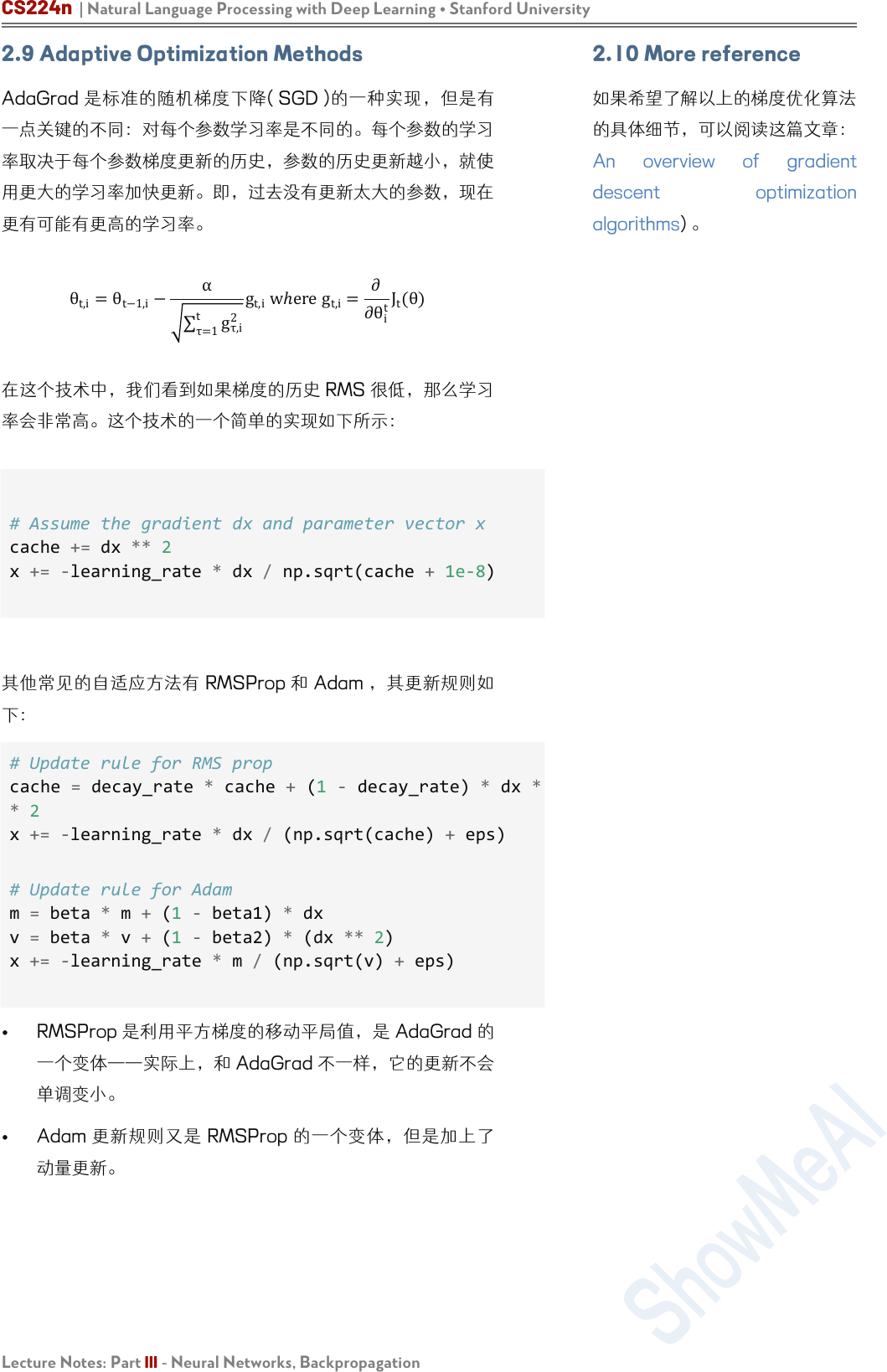CS224n | Natural Language Processing with Deep Learning Stanford University
Lecture Notes: Part III - Neural Networks, Backpropagation

θ
t,i
=
θ
t−1,i
α
τ=1
t
g
τ,i
2
g
t,i
wℎere g
t,i
=
∂θ
i
t
J
t
(θ)

# Assume the gradient dx and parameter vector x
cache += dx ** 2
x += -learning_rate * dx / np.sqrt(cache + 1e-8)

# Update rule for RMS prop
cache = decay_rate * cache + (1 - decay_rate) * dx *
* 2
x += -learning_rate * dx / (np.sqrt(cache) + eps)
m = beta * m + (1 - beta1) * dx
v = beta * v + (1 - beta2) * (dx ** 2)
x += -learning_rate * m / (np.sqrt(v) + eps)

2.10 More reference

descent optimization
algorithms)Awesome AI Courses Notes Cheat Sheets
@
ShowMeAI
Lecture Notes: Part III - Neural Networks, Backpropagation

Machine Learning
Deep Learning
Natural Language Processing
Computer Vision
Knowledge Graphs
Stanford · CS229
Stanford · CS230
Stanford · CS224n
Stanford · CS231n
Stanford · CS520
# 系列内容 Awesome AI Courses Notes Cheatsheets

Machine Learning with Graphs
Deep Reinforcement Learning
Deep Learning for Self-Driving Cars
Stanford · CS224W
UCBerkeley · CS285
MIT · 6.S094
...
...
...CS224n | Natural Language Processing with Deep Learning Stanford University
21
ShowMeAI 的分覆盖知名TOP20+ AI 旨在学习一整